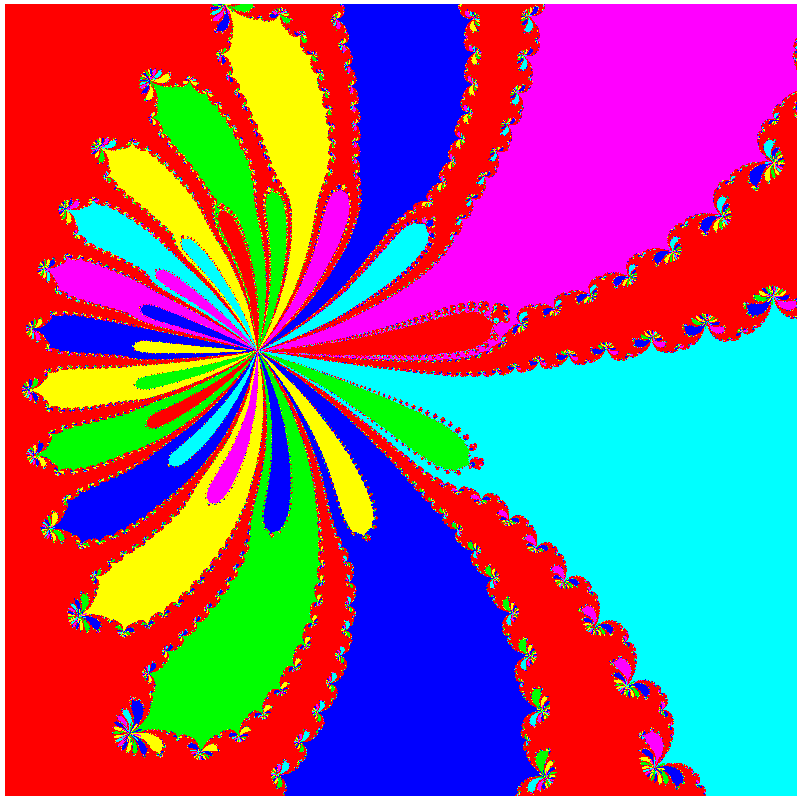Newton iteration in the complex plane for a polynomial of degree 32 whose zeros are: 0, minus the 15th roots of the unity and two times the 16th roots of the unity. The figure displays a colour code according to the zero to which Newton iteration, starting at the plotted point, converges. Note that different zeros can have the same colour assigned. Iterations are stopped when an iterate is at a distance less than 0.01 from one zero. Left lower corner: (0.732923563,1.76942831). Horizontal and vertical width: 1E-8. 800 x 800 pixels are used. Estimated number of arithmetic floating point double precision operations: 70.90E9. Total (manual cronometer) time: 31 seconds.